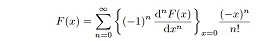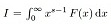##### Some Applications of Celebrated Master Theorem of Ramanujan

M. I. Qureshi, Kaleem A. Quraishi, Ram Pal

Journal of Advances in Mathematics and Computer Science, Page 2862-2871
DOI: 10.9734/BJMCS/2014/4842

If F(x) is expanded in the form of Maclaurin’s seriesthen Ramanujan asserts that the value ofcan be found from the coefficient

ofin the expansion of F(x). Conversely Ramanujan claims that if the value of I is known,
then the Maclaurin’s coefficient of F(x) can be found.
In this paper, we obtain Maclaurin’s expansions and Mellin transforms of some composite functions,
using Ramanujan’s Master theorem.

##### Dynamic Video Texture Inpainting Using Improving LDS

Mohsen Soryani, Amanna Ghanbari, Abbas Koochari

Journal of Advances in Mathematics and Computer Science, Page 2872-2883
DOI: 10.9734/BJMCS/2014/4751

Inpainting or completion is used with the aim of restoring damaged regions in images and video frames using the safe regions. This paper introduces a novel approach based on LDS (Linear Dynamic Systems) for inpainting of corrupted video frames which include dynamic textures. In this work, a mask is defined for each frame corresponding to the damaged portion of the frame; the mask determines the target region that will be completed by the proposed method. Notice that the mask is moving. To verify whether corrupted frames are reconstructed correctly or not, a measure named MS-SSIM (Multi-Scale Structural Similarity) is used. The value close to one for this scale introduces more similarity between the two components that are going to be compared. The obtained value for the above mentioned measure is very close to one for our results and the generated video is pleasant.

##### Computing Power Utilization of Distributed Systems Using Distributed Compilation: A Clustered HPC Approach

Sunil Kr Singh, Aman Madaan, Ankur Aggarwal, Ankur Dewan

Journal of Advances in Mathematics and Computer Science, Page 2884-2900
DOI: 10.9734/BJMCS/2014/10885

To implement a farm of computer system which compiles source programs using the computers connected in the farm. A build farm can be defined as a set of computers (distributed systems) that work together to compile software. Distributed Compiling is a special type of compiling activity in which we compile the source program using several machines with the same or cross compilers to do the compilation in parallel. In this paper, we describe a system that uses the concept for reducing build times by using free cycles of idle computer systems in distributed. The challenge of distributing compilation is tackled by a distributing compiler. We use distcc for the purpose but It would be nice if distcc could automatically detect the best distribution, but it doesn't do that yet. But in our system we have added a new php script which automatically does this work. Whenever it sees that a system is overloaded or its utilization increases above a threshold value (in our case it is 65%), it automatically removes that node. In this way we can combine the computing powers of individual machines to get a more powerful machine.

##### Statistical Texture and Normalized Discrete Cosine Transform-Based Automatic Soya Plant Foliar Infection Cataloguing

Sourabh Shrivastava, Satish Kumar Singh, Dhara Singh Hooda

Journal of Advances in Mathematics and Computer Science, Page 2901-2916
DOI: 10.9734/BJMCS/2014/11973

Soya beans are an important cash-crop, grown worldwide because of their use as a raw material in various industries involved in the production of sauces, mayonnaises, chocolates, baby-food, bakery, etc. Soya bean products are not only consumed by humans, but by pets too. They are also used as an alternative to fossil fuel in the form of bio-diesel. The research work presented in this paper highlights the problems associated with soya bean cultivation, and the reasons for yield loss in developing countries like India, China and others. In this paper, the colour image sensing and processing based infected lesion detection method is proposed. Structural texture and normalized DCT-based feature descriptors for refined lesion histograms have been developed. Hybrid feature descriptors have been used as the input samples for four major soya plant foliar infections. The classification and testing accuracies are quite encouraging, and are above 89.9% and 92.3% respectively. Also, the results have been compared for only ST, ST-DCT, and ST-NDCT feature-based methods, and the use of ST-NDCT descriptors in cataloguing systems is suggested. Moreover, the method can classify four diseases, which creates confusion because of similar colour shades, and irregular and random shapes and sizes of lesions.

##### An Approximate Sequential Bundle Method for Solving a Convex Nondifferentiable Bilevel Programming Problem

Jie Shen, Li-Ping Pang, Xi-Jun Liang, Zun-Quan Xia

Journal of Advances in Mathematics and Computer Science, Page 2917-2928
DOI: 10.9734/BJMCS/2014/12022

By combining two bundle methods, PBMASL (proximal bundle method with approximate subgradient linearizations) and DPLBM (descent proximal level bundle method), we present an approximate sequential bundle algorithm for solving a bilevel programming problem with a nondifferentiable convex objective function and two separable constraints. In the proposed algorithm, the values of the objective function in the constraints and its subgradients are computed approximately, the estimates of the tolerances are not required for convergence proof. The presented results improve and extend the earlier work.

##### Some Contributions to the Solution of Cubic Equations

O. E. Okereke, I. S. Iwueze, J. Ohakwe

Journal of Advances in Mathematics and Computer Science, Page 2929-2941
DOI: 10.9734/BJMCS/2014/10934

In many applications, the solution of a cubic equation is required. This study improves on the existing methods of solving cubic equations. The general expression for the quadratic factor of a cubic equation is derived. The discriminant of the cubic equation, adopted in this study as D, comprises of two terms, D1 and D2 such that D = D12 - D23 and the value of D is shown to depend on the nature of the root of a given cubic equation. For a cubic equation to have three distinct real roots, D<0. If  D>0, the equation has only one real root, and if D = 0, the equation has either two equal real roots or three equal real roots. A required and sufficient condition for a cubic equation to have three equal real roots is shown to be D= 0, D2 = 0 and D = 0. It is also established that a cubic equation has two real equal roots if D1 ≠ 0, D2 ≠ 0, but D12 =  D23 and D = 0. The solution of a cubic equation with three real equal roots is found using a method that depends on the corresponding reduced cubic equation. Finally, numerical examples are used to demonstrate the established results.

##### Analysis of an SI Epidemic Model with Nonlinear Incidence Rate in an Environmentally-driven Infectious Disease

Mimi Yan, Ruiqing Shi

Journal of Advances in Mathematics and Computer Science, Page 2942-2953
DOI: 10.9734/BJMCS/2014/11768

In this paper, a new model for the linking of within- and between-host with nonlinear incidence rate is constructed and investigated. We postulate that the infection process depend on the size of the infective inoculum that susceptible hosts may acquire by interacting with a contaminated environment. Because the dynamical processes associated with the within- and between-host occur on different time scales, the model behaviors can be analyzed by using a singular perturbation argument, which allows us to decouple the full model by separating the fast- and slow-systems. For the fast system, it is shown that the infection-free equilibrium Uο is locally asymptotically stable if Rvο < 1; whereas Uο is unstable if Rvο > 1, and a unique interior equilibriumexists and it is locally asymptotically stable. For the slow system, under the condition that the fast system has a stable interior equilibrium( i.e., Rvο > 1), there exists at least one endemic equilibrium W if Rh > 1 and βN < 1. We also prove that the endemic equilibrium W for the slow system is locally asymptotically stable if R*hο > 1. Some numerical simulations are provided to verify the theoretical results. In addition, some simple discussion is also presented at the end of the article.

##### Existence and Uniqueness of Solution to Nuclear Safety Characterization Associated with Penalized Quadratic Cost Functional

L. Aminu, J. O. Omolehin

Journal of Advances in Mathematics and Computer Science, Page 2954-2959
DOI: 10.9734/BJMCS/2014/11046

The residual parametric equation of the heat equations of nuclear reactors were transformed into control system. Optimization techniques were used to established the existence and uniqueness of the solution to the model.

##### Complete Synchronization of Two Bidirectionally Coupled Piecewise Linear Chaotic Systems Perturbed by Poisson Noise

Bin Pei, Yong Xu

Journal of Advances in Mathematics and Computer Science, Page 2960-2977
DOI: 10.9734/BJMCS/2014/12578

We establish the sufficient conditions of complete synchronization with probability 1 in the coupled bidirectional piecewise linear chaotic systems with common or different Poisson noises and the positive effects of Poisson noises on the complete synchronization will be found based on the proposed theoretical results. We compare the effects of Poisson noise on the complete synchronization with the case of Gaussian white noise, and then we claim Poisson noise can induce complete synchronization easier than Gaussian white noise in the sense of noise strength. Furthermore, a numerical example is presented to show the excellent agreement between theoretical and numerical analysis.

##### Genetic Algorithm and Rough Sets Based Hybrid Approach for Economic Environmental Dispatch of Power Systems

Mohamed A. Hussein, Ahmed A. EL-Sawy, EL-Sayed M. Zaki, A. A. Mousa

Journal of Advances in Mathematics and Computer Science, Page 2978-2999
DOI: 10.9734/BJMCS/2014/11526

In this paper we present a new optimization algorithm for Economic environmental dispatch EED of power systems. The purpose of EED problem is to compute the optimal generation for individual units of the power system by minimizing the fuel cost and emission levels simultaneously, subject to various equality and inequality constraints. The proposed algorithm is population based an evolutionary algorithm which operates in two phases: in the first one, genetic algorithm is implemented as search engine in order to generate approximate true Pareto front. This algorithm based on concept of co-evolution and repair algorithm for handling nonlinear constraints. Also it maintains a finite-sized archive of non-dominated solutions which gets iteratively updated in the presence of new solutions based on the concept of ε -dominance. Then, in the second phase, rough sets theory is adopted as local search engine in order to improve the spread of the solutions found so far. Optimization using multiobjective evolutionary algorithms yields not a single optimal solution. However, for practical applications, we need to select one solution which will satisfy the different goals to some extent. TOPSIS method has the ability to identify the best alternative from a finite set of alternatives. The proposed approach is carried out on the standard IEEE 30-bus 6-generator test system. The results demonstrate the capabilities of the proposed approach to generate true and well-distributed Pareto-optimal nondominated solutions of the multiobjective EED problem. Also the comparison with the exiting well-known algorithms demonstrates the superiority of the proposed approach and confirms its potential to solve the multiobjective EED problem.